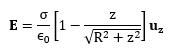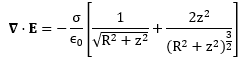# Doubt about the 1st Law of Maxwell

Good afternoon,

My question concerns Maxwell's first law. Knowing the formula of the electric field created by a uniformly charged disk, we calculate its divergence and evaluate it at one point, it does not give us zero, as it should be because the volumetric density of charge is zero, why does this happen? Where is the error in my reasoning?

## Answers and Replies

BvU
Science Advisor
Homework Helper
we calculate its divergence and evaluate it at one point, it does not give us zero, as it should
Please post your case !

•vanhees71
Dale
Mentor
2020 Award
we calculate its divergence and evaluate it at one point, it does not give us zero, as it should be because the volumetric density of charge is zero, why does this happen? Where is the error in my reasoning?
In the disk the charge density will not be zero. Outside the disk the charge density will be zero. If you violate Gauss' law anywhere then you made a math error.

The electric field due to a disk of radius R on the z axis is:And its divergence will be:That evaluated at a point other than z = 0 is not necessarily zero, I know there has to be an error in my reasoning but I am not able to find it.

## \nabla \cdot \mathbf{E} = \frac{\rho}{\epsilon_0} ##
Is the most common form because in real life we get volume charge distributions. See, this is how we find electric field for volume distribution of charges $$\mathbf{E} = \frac{1}{4\pi \epsilon_0} \int_V \frac{\rho (\vec r)}{r^2} dV$$ but you must have seen (or used) this form $$\mathbf{E} = \frac{1}{4\pi \epsilon_0} \int_L \frac{\lambda (\vec r)}{r^2} dl$$ So, for the case that you have posted we need to write the 1st equation of Electrostatics as $$\nabla \cdot \mathbf E = \frac{\sigma}{\epsilon_0}$$

Okay, but still ... at points outside the disk, the surface charge density is zero, but according to my calculations the divergence of E is not ...

Okay, but still ... at points outside the disk, the surface charge density is zero, but according to my calculations the divergence of E is not ...
Take any closed surface not containing the disk, then $$\oint_S \vec E \cdot d\vec a = Q_{enclosed}/ \epsilon_0$$ since no charge is contained therefore the right side is zero, $$\oint_S \vec E \cdot d\vec a = 0$$ But by divergence theorem we can write that $$\int_V (\nabla \cdot \vec E ) dV = \oint_S \vec E \cdot d\vec a \\ \int_V (\nabla \cdot \vec E ) dV =0 \\ \nabla \cdot \vec E= 0$$

Yes, I agree that I should give zero, what happens is that calculating the divergence of the field (from the expressions I left above), does not give zero ...

Yes, I agree that I should give zero, what happens is that calculating the divergence of the field (from the expressions I left above), does not give zero ...
That’s not working because the charge density is zero everywhere but suddenly reach to ##\sigma## as we enter the disk and hence we need delta function for that. You try to to calculate the divergence of an electric field of a single point charge then I assure you that you will get zero for that everywhere. But it should not be zero at the place where the point charge is.

When you calculated the divergence in your earlier post you took ##\sigma## as constant but ## \frac{\partial \sigma}{\partial x} ## is not zero, ##\sigma## is varying (abruptly from the disk to the outside of it)

Last edited by a moderator:
mjc123
Science Advisor
Homework Helper
∇⋅E = dEx/dx + dEy/dy + dEz/dz
On the z axis, Ex and Ey are zero, but dEx/dx and dEy/dy are not.

•beamie564, BvU, jtbell and 1 other person
Dale
Mentor
2020 Award
The electric field due to a disk of radius R on the z axis is:
You cannot calculate divergence from a formula that is restricted to the z axis.

You need to use a formula for the electric field throughout space, not just on the z axis.

jtbell
Mentor
∇⋅E = dEx/dx + dEy/dy + dEz/dz
On the z axis, Ex and Ey are zero, but dEx/dx and dEy/dy are not.
To see this more explicitly, consider the simpler example of a point charge at the origin. Calculate the partial derivatives for a general point (x,y,z), then set x = y = 0 for a point on the z-axis.

ZapperZ
Staff Emeritus
Science Advisor
Education Advisor
Okay, but still ... at points outside the disk, the surface charge density is zero, but according to my calculations the divergence of E is not ...

That expression that you used is ONLY VALID along the z-axis! You cannot use that as the only field for all space!

That is the most obvious error in your calculation.

Zz.

•vanhees71
vanhees71
Science Advisor
Gold Member
The electric field due to a disk of radius R on the z axis is:

View attachment 255645
And its divergence will be:

View attachment 255646
That evaluated at a point other than z = 0 is not necessarily zero, I know there has to be an error in my reasoning but I am not able to find it.
You cannot evaluate the divergence without knowing the electric field everywhere!

BvU
Science Advisor
Homework Helper
@Cloruro de potasio : is it clear to you now that you will need ##{\partial E\over \partial x}## and ##{\partial E\over \partial y}## at your chosen point (where they are equal) and add them to ##{\partial E\over \partial z}## to get 0 ?

vanhees71
Science Advisor
Gold Member
It's
$$\vec{\nabla} \cdot \vec{E}=\partial_x E_x + \partial_y E_y + \partial_z E_z.$$

•BvU